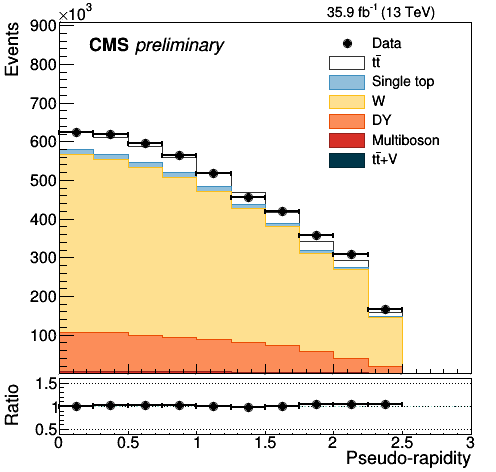# How to stack histograms for signals and background

Dear Experts
I have a problem regarding stacking of histograms. I want to stack histograms for signals and backgrounds. I am getting my variables (Histograms) from muminus_plotter.root. When i try to draw it give me blank plots with no data. The code as well as the result is given below. Kindly if you can help me to figure it out.

Code

``````{
{
TString path = "/root/from_Qamar/";

TFile *file1 = new TFile(path+"muminus_plotter.root");

THStack hs("hs"," stacked histograms");

TH1F *h_0 = new TH1F("h_0","h_0",100,-100,100);
TH1F *h_1 = new TH1F("h_1","h_1",100,-100,100);
TH1F *h_3 = new TH1F("h_3","h_3",100,-100,100);
TH1F *h_4 = new TH1F("h_4","h_4",100,-100,100);
TH1F *h_5 = new TH1F("h_5","h_5",100,-100,100);

TH1F* h_0 = (TH1F*)file1->Get("nbtags_4j/nbtags_4j_W");
TH1F* h_1 = (TH1F*)file1->Get("nbtags_4j/nbtags_4j_DY");
TH1F* h_3 = (TH1F*)file1->Get("nbtags_4j/nbtags_4j_Single top");
TH1F* h_4 = (TH1F*)file1->Get("nbtags_4j/nbtags_4j_Multiboson");
TH1F* h_5 = (TH1F*)file1->Get("nbtags_4j/nbtags_4j_t#bar{t}+V");

//signal
TH1F* h_2 = (TH1F*)file1->Get("nbtags_4j/nbtags_4j_t#bar{t}");
TH1F *h_2 = new TH1F("h_2","h_2",100,-100,100);
h_0->Fill("nbtags_4j/nbtags_4j_W",1000);
h_0->SetFillColor(kRed);
h_0->GetYaxis()->SetRange(0,100);
h_0->GetXaxis()->SetRange(0,100);
h_0->Draw();

h_1->Fill("nbtags_4j/nbtags_4j_DY",1000);
h_1->SetFillColor(kBlue);

h_3->FillRandom("nbtags_4j/nbtags_4j_Single top",2000);
h_3->SetFillColor(kGreen);

h_4->FillRandom("nbtags_4j/nbtags_4j_Multiboson",3000);
h_4->SetFillColor(kBlack);

h_5->FillRandom("nbtags_4j/nbtags_4j_t#bar{t}+V",7000);
h_5->SetFillColor(kYellow);

hs->Draw("stack");
TCanvas*c1=new TCanvas("c1","stacked hists",700,900);
c1->Divide (1,2);
c1->cd(1);
hs->Draw();
c1->cd(2);
hs->Draw("stack");
}
``````

Faiza Naveed

Actually i want to obtain the final result like given below in the imageI would suggest you do only:

``````hs->Draw();
``````

``````hs->Draw("stack");
TCanvas*c1=new TCanvas("c1","stacked hists",700,900);
c1->Divide (1,2);
c1->cd(1);
hs->Draw();
c1->cd(2);
hs->Draw("stack");
``````

This is a minimally working example

``````void test() {
THStack * hs1 = new THStack("hs1"," stacked");
THStack * hs2 = new THStack("hs2"," unstacked");

TH1F *h_0 = new TH1F("h_0","h_0",100,-10,10);
TH1F *h_1 = new TH1F("h_1","h_1",100,-10,10);

//signal
h_0->FillRandom("gaus",1000);
h_0->SetFillColor(kRed);

h_1->FillRandom("gaus",500);
h_1->SetFillColor(kBlue);

TCanvas * c1 = new TCanvas("c1","stacked hists",700,900);
c1->Divide(1, 2);
c1->cd(1);
hs1->Draw("");
c1->cd(2);
hs2->Draw("nostack");
}
``````

This biggest difference here, besides the way the data is generated, is found on the first line. I switched:
`THStack hs("hs"," stacked histograms");`
for
`THStack * hs1 = new THStack("hs1"," stacked");`

Apparently root doesn’t play well with stack variables. I suspect that in you example hs goes out of scope when the function returns, so when the canvas is painted the histograms are gone already.

This topic was automatically closed 14 days after the last reply. New replies are no longer allowed.